• 二行二列矩阵计算公式
千次阅读
2021-10-28 15:01:08

## 前言

熟练使用python计算向量、矩阵、数组之后，是不是就不用matlab了？应该不是，matlab的函数完善，而python我还不知道缺什么函数和功能。

## 基本操作

### 创建

import numpy as np
#创建行向量[1,2,3]
print(np.array([1,2,3]))

#创建列向量[,,]
print(np.array([,,]))

#创建矩阵
print(np.array([[1,2,3],[4,5,6],[7,8,9]]))

#创建矩阵
print(np.mat([[1,2,3],[4,5,6],[7,8,9]])) #不推荐，因为大多数操作都是数组而不是矩阵数据结构mat

#-------创建三维矩阵-------

#创建1个2X3的0矩阵
print('>',np.zeros((1,2,3)))

#创建两个2X2的0矩阵
print('>',np.zeros((2,2,2)))

#创建两个3X3的1矩阵
print('>>>',np.ones((2,3,3)))

#-----------------------

from scipy import sparse
#创建稀疏矩阵
matrix =np.array([[0,0,0,1],[0,2,0,1],[1,0,3,0]])
#创建一个压缩的稀疏行矩阵compressed sparse row
print(sparse.csr_matrix(matrix))

[1 2 3]
[

]
[[1 2 3]
[4 5 6]
[7 8 9]]
[[1 2 3]
[4 5 6]
[7 8 9]]
> [[[0. 0. 0.]
[0. 0. 0.]]]
> [[[0. 0.]
[0. 0.]]

[[0. 0.]
[0. 0.]]]
>>> [[[1. 1. 1.]
[1. 1. 1.]
[1. 1. 1.]]

[[1. 1. 1.]
[1. 1. 1.]
[1. 1. 1.]]]
(0, 3)	1
(1, 1)	2
(1, 3)	1
(2, 0)	1
(2, 2)	3


### 选择元素

import numpy as np
from scipy import sparse
#创建行向量
v = np.array([1,2,3,4,5,6])
#创建矩阵
m = np.array([[1,2,3],
[4,5,6],
[7,8,9]])

#第5个
print(v[5-1])
#第三行第二个元素
print(m[3-1][2-1])
print(m[2,1])

#选取向量所有元素
print(v[:])

#选取0到第三个
print(v[:3])

#选取第三个到最后
print(v[3:])

#选取最后一个
print(v[-1])

#选取矩阵全部
print(m[:])
print(m)

#选取矩阵第一行
print(m[0,:])

#选取第一行和第三行
print(m[0,:],m[2,:])

#选取第二列
print(m[:,1])

5
8
8
[1 2 3 4 5 6]
[1 2 3]
[4 5 6]
6
[[1 2 3]
[4 5 6]
[7 8 9]]
[[1 2 3]
[4 5 6]
[7 8 9]]
[1 2 3]
[1 2 3] [7 8 9]
[2 5 8]


### 矩阵的属性

import numpy as np
from scipy import sparse
m = np.array([[1,2,3,4],
[5,6,7,8],
[9,10,11,12]])

#行，列
a = m.shape
print(a)
print('r=%d,c=%d'%(a,a))

#元素数量
print(m.size)

#矩阵的维数
print(m.ndim)

(3, 4)
r=3,c=4
12
2


虽然矩阵有4列，但是因为4个基向量是线性相关的，最大线性无关组只有两个，维数也就只有两个。

### 矩阵的秩

import numpy as np
from scipy import sparse
#创建矩阵
m = np.array([[1,2,3,0],
[4,5,6,0],
[7,8,9,0]])

print(np.linalg.matrix_rank(m))

2


### 矩阵的迹

import numpy as np
from scipy import sparse
#创建矩阵
m = np.array([[1,2,3,0],
[4,5,6,0],
[7,8,9,0]])

print(m.trace())

15


### vectorize

import numpy as np
from scipy import sparse
#创建行向量
v = np.array([1,2,3,4,5,6])
#创建矩阵
m = np.array([[1,2,3],
[4,5,6],
[7,8,9]])

#创建一个向量化的函数
func = np.vectorize(lambda x:x+100)  #vectorize把一个函数变成另一个函数
#对所有元素应用这个函数
print(func(m))

#直接操作也行
print(m-100)

print(m*2)

print(m/2)


[[101 102 103]
[104 105 106]
[107 108 109]]
[[-99 -98 -97]
[-96 -95 -94]
[-93 -92 -91]]
[[ 2  4  6]
[ 8 10 12]
[14 16 18]]
[[0.5 1.  1.5]
[2.  2.5 3. ]
[3.5 4.  4.5]]


### 最值、平均值、方差、标准差

import numpy as np
from scipy import sparse
#创建矩阵
m = np.array([[1,2,3],
[4,5,6],
[7,8,9]])

print(np.max(m))
print(np.min(m))
print(np.mean(m))
print(np.var(m))
print(np.std(m))

9
1
5.0
6.666666666666667
2.581988897471611


### 矩阵变形

import numpy as np
from scipy import sparse
#创建矩阵
m = np.array([[1,2,3,0],
[4,5,6,0],
[7,8,9,0]])

#变形前后元素个数一样
print(m.reshape(2,6))

[[1 2 3 0 4 5]
[6 0 7 8 9 0]]


### 转置

import numpy as np
from scipy import sparse
#创建行向量
v = np.array([1,2,3,4,5,6,7,8,9])
#创建矩阵
m = np.array([[1,2,3,0],
[4,5,6,0],
[7,8,9,0]])

print(m.T)

#向量只是值的集合，不能转置
print(v)
print(v.T)
print(np.array([1,2,3,4,5,6,7,8,9]).T)

[[1 4 7]
[2 5 8]
[3 6 9]
[0 0 0]]
[1 2 3 4 5 6 7 8 9]
[1 2 3 4 5 6 7 8 9]
[1 2 3 4 5 6 7 8 9]


### 矩阵展开

import numpy as np
from scipy import sparse
#创建矩阵
m = np.array([[1,2,3,0],
[4,5,6,0],
[7,8,9,0]])

print(m.flatten())

[1 2 3 0 4 5 6 0 7 8 9 0]


## 高级操作

### 行列式

import numpy as np
from scipy import sparse
#创建矩阵
m = np.array([[1,2,3,0],
[4,5,6,0],
[7,8,9,0],
[0,0,0,0]])

print(np.linalg.det(m))

0.0


### 对角线元素

import numpy as np
from scipy import sparse
#创建矩阵
m = np.array([[1,2,3,0],
[4,5,6,0],
[7,8,9,0] ])

print(m.diagonal())

[1 5 9]


### 特征值和特征向量

import numpy as np
from scipy import sparse
#创建矩阵
m = np.array([[1,2,3,7],
[4,5,6,0],
[7,8,9,0],
[0,0,1,4]])

#特征值和特征向量
a,b = np.linalg.eig(m)

print(a)
print('-------------')
print(b)

[16.33839194+0.j         -0.29035096+0.55867363j -0.29035096-0.55867363j
3.24230999+0.j        ]
-------------
[[-0.25665901+0.j         -0.69644039+0.j         -0.69644039-0.j
0.70851102+0.j        ]
[-0.52018868+0.j          0.14699415-0.42878781j  0.14699415+0.42878781j
-0.35480461+0.j        ]
[-0.81191118+0.j          0.37461056+0.39175996j  0.37461056-0.39175996j
-0.36840126+0.j        ]
[-0.06580365+0.j         -0.07416678-0.10096959j -0.07416678+0.10096959j
0.48621634+0.j        ]]



### 点积和叉积

import numpy as np
from scipy import sparse

a = np.array([1,2,3,4])
b = np.array([11,2,3,4])
print(np.dot(a,b))

c = np.array([[34,2],
[1,3]])
d = np.array([[2,9],
[0,1]])
print(np.cross(c,d)) #cross必须是两个二维矩阵相叉乘，或两个三维矩阵相叉乘

e = np.array([[1,2],
[1,0]])
f = np.array([[3,5],
[1,2]])
print(np.cross(e,f))

40
[302   1]
[-1  2]


两个二维矩阵叉乘公式
A = [ a 11 a 12 a 21 a 22 ] A = \left[ \begin{matrix} a_{11} \quad a_{12} \\ a_{21} \quad a_{22} \\ \end{matrix}\\ \right]
B = [ b 11 b 12 b 21 b 22 ] B = \left[ \begin{matrix} b_{11} \quad b_{12} \\ b_{21} \quad b_{22} \\ \end{matrix} \right]

A × B = [ a 11 b 12 − a 12 b 11 a 21 b 22 − a 22 b 21 ] A\times B = \left[ \begin{matrix} a_{11}b_{12} - a_{12}b_{11} \qquad a_{21}b_{22} - a_{22}b_{21}\end{matrix}\right]

### 矩阵的逆

import numpy as np
from scipy import sparse
#创建矩阵
m = np.array([[1,2,3,7],
[4,5,6,4],
[7,8,9,2],
[1,3,12,5]])

print(np.linalg.inv(m))

[[ 8.42857143e+00 -2.01428571e+01  1.04285714e+01  1.42857143e-01]
[-8.85714286e+00  2.12857143e+01 -1.08571429e+01 -2.85714286e-01]
[ 1.09523810e+00 -2.80952381e+00  1.42857143e+00  1.42857143e-01]
[ 1.00000000e+00 -2.00000000e+00  1.00000000e+00  1.70803542e-17]]


### 生成随机数

import numpy as np

#设置随机数种子
np.random.seed(0)

#生成3个0到1的数
print(np.random.random(3))  #默认0~1

#生成3个1到100的整数
print(np.random.randint(0,101,3))

#从1<=x<2 中抽3个
print(np.random.uniform(1,2,3))

#正态分布
#平均值0，标准差1
print(np.random.normal(0,1,3))

#逻辑分布
#平均值0，散布程度1
print(np.random.logistic(0,1,3))

[0.5488135  0.71518937 0.60276338]
[67  9 83]
[1.64589411 1.43758721 1.891773  ]
[-0.10321885  0.4105985   0.14404357]
[ 0.27387744  2.52093742 -2.57088231]机器学习
更多相关内容
• 矩阵中的数据按排列与按排列求出的协方差矩阵是不同的，这里默认数据是按排列。即每一是一个observaTIon(or sample)，那么每一就是一个随机变量。协方差矩阵：协方差矩阵的维度等于随机变量的个数，即每一...

协方差矩阵是什么

在统计学与概率论中，协方差矩阵的每个元素是各个向量元素之间的协方差，是从标量随机变量到高维度随机向量的自然推广。

矩阵中的数据按行排列与按列排列求出的协方差矩阵是不同的，这里默认数据是按行排列。即每一行是一个observaTIon(or sample)，那么每一列就是一个随机变量。协方差矩阵：协方差矩阵的维度等于随机变量的个数，即每一个 observaTIon 的维度。在某些场合前边也会出现 1 / m，而不是 1 / (m - 1)。

协方差矩阵计算公式

在统计学与概率论中，协方差矩阵是一个矩阵，其每个元素是各个向量元素之间的协方差。这是从标量随机变量到高维度随机向量的自然推广。如何计算协方差矩阵

举个例子，矩阵 X 按行排列：1. 求每个维度的平均值2. 将 X 的每一列减去平均值其中：3. 计算协方差矩阵注意：

有时候在书上或者网上会看到这样的公式，协方差矩阵 Σ：这里之所以会是 X * X‘ 是因为原始数据集 X 是按列排列的，即：另外在 opencv 文档中关于计算协方差矩阵的 calcCovarMatrix 函数的说明我觉得是有问题的，

在正常的 CV_COVAR_NORMAL 状态下它给出的 cov = scale * X * X’，而这个定义仅仅是在数据按列排列下才有的，即 CV_COVAR_COLS.

URL：http://docs.opencv.org/modules/core/doc/operaTIons_on_arrays.html？highlight=calccovarmatrix#cv.CalcCovarMatrix

这里有一个按列排列的 PCA 教程，可以参考一下：

http://ufldl.stanford.edu/wiki/index.php/%E4%B8%BB%E6%88%90%E5%88%86%E5%88%86%E6%9E%90

matlab 代码：

clear; clc;

% data are stored as rows.

X = ［1 2 3;

3 1 1］;

Y = X;

［rows， cols］ = size(X);

% get mean of each dimension(each column)。

meanMatrix = mean(X);

% X - mean.

X = X - ones(rows， 1) * meanMatrix;

% get the cov matrix.

covMatrix = 1 / (rows - 1) * (X‘ * X)

% the given ’cov‘ funcTIon

cov(Y)

展开全文• 矩阵相乘的前提条件是：乘号前的矩阵数要和乘号后的矩阵的行数...计算方法是：用矩阵A的第 i 矩阵B中的每一 j 对应的数值做乘法运算，乘积一一相加，所得结果即为矩阵 C 中第 i 第 j 的值。得到的乘积...

矩阵相乘的前提条件是：乘号前的矩阵的列数要和乘号后的矩阵的行数相等。且矩阵的乘法运算没有交换律，即 A*B 和 B*A 是不一样的。

例如，矩阵A：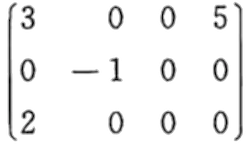矩阵B：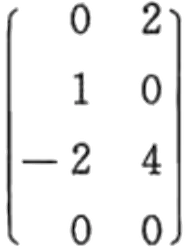由于矩阵 A 的列数和矩阵 B 的行数相等，可以进行 A*B 运算(不能进行 B*A 运算)。计算方法是：用矩阵A的第 i 行和矩阵B中的每一列 j 对应的数值做乘法运算，乘积一一相加，所得结果即为矩阵 C 中第 i 行第 j 列的值。

得到的乘积矩阵C为：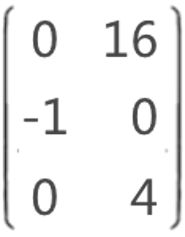例如：C12= 6 是因为：A11*B12+ A12*B22+ A13*B32+ A14*B42，即 3*2 + 0*0 + 0*4 + 5*0 = 6 ，因为这是 A 的第 1 行和 B 的第 2 列的乘积和，所以结果放在 C 的第 1 行第 2 列的位置。

例如，A是 m1*n1 矩阵，B是 m2*n2 矩阵(前提必须是 n1 == m2 )：

int C[MAX][MAX];

for (int i=0; i

for (int j=0; j

C[i][j]=0;

for (int k=0; k

C[i][j]+=A[i][k]*B[k][j];

}

}

}

普通算法的O(m1*n2*n1)。

在稀疏矩阵做乘法运算时，由于本身矩阵中含有的非 0 元素少，普通算法会出现很多 0*0 或者 k*0 或者 0*k ( k 代表非 0 元素值)的情况。下面介绍使用

行逻辑链接的

对矩阵的乘积进行深度剖析，矩阵 A 和矩阵 B 相乘的运算过程是这样的：

首先，找到矩阵 A 中第一行的非 0 元素，分别是 A11 = 3和 A14 = 5；(由于行逻辑链接的顺序表中存储的都是非 0 元素，查找的过程就需要使用记录每行第一个非 0 元素的首地址的

用 3 去和 B 中对应的第一行中的非 0 元素相乘，矩阵 B 中第一行非 0 元素是 B12 = 2，所以 3*2 = 6 ，因为 6 是 A11和 B12相乘的结果，所以暂时存放在 C12中；用 5 去和 B 中对应的第 4 行的非 0 元素相乘，由于矩阵 B 中第 4 行没有非 0 元素，所以，第一行的计算结束；

以此类推。

攻克问题难点

现在，解决问题的关键在于，如何知道顺序表中存放的非0元素是哪一行的呢？

解决方案：由于使用的是行逻辑链接的顺序表，所以，已经知道了每一个矩阵中的每一行有多少个非0元素，而且第一行的第一个非0元素的位置一定是1。

所以，第 n 行的非0元素的位置范围是：大于或等于第 n 行第一个元素的位置， 小于第 n+1 行第一个元素的位置(如果是矩阵的最后一行， 小于矩阵中非 0 元素的个数 + 1)。

具体实现代码

#include

#define MAXSIZE 12500

#define MAXRC 100

#define ElemType int

typedef struct

{

int i,j;//行，列

ElemType e;//元素值

}Triple;

typedef struct

{

Triple data[MAXSIZE+1];

int rpos[MAXRC+1];//每行第一个非零元素在data数组中的位置

int mu,nu,tu;//行数，列数，元素个数

}RLSMatrix;

RLSMatrix MultSMatrix(RLSMatrix A, RLSMatrix B, RLSMatrix C)

{

//如果矩阵A的列数与矩阵B的行数不等，则不能做矩阵乘运算

if(A.nu != B.mu)

return C;

C.mu = A.mu;

C.nu = B.nu;

C.tu = 0;

//如果其中任意矩阵的元素个数为零，做乘法元素没有意义，全是0

if(A.tu * B.tu == 0)

return C;

else

{

int arow;

int ccol;

//遍历矩阵A的每一行

for(arow=1; arow<=A.mu; arow++)

{

//创建一个临时存储乘积结果的数组，且初始化为0，遍历每次都需要清空

int ctemp[MAXRC+1] ={};

C.rpos[arow] = C.tu + 1;

//根据行数，在三元组表中找到该行所有的非0元素的位置

int tp;

if(arow < A.mu)

tp = A.rpos[arow+1];//获取矩阵A的下一行第一个非零元素在data数组中位置

else

tp = A.tu+1;//若当前行是最后一行，则取最后一个元素+1

int p;

int brow;

//遍历当前行的所有的非0元素

for(p=A.rpos[arow]; p

{

brow = A.data[p].j;//取该非0元素的列数，便于去B中找对应的做乘积的非0元素

int t;

// 判断如果对于A中非0元素，找到矩阵B中做乘法的那一行中的所有的非0元素

if(brow < B.mu)

t = B.rpos[brow+1];

else

t = B.tu+1;

int q;

//遍历找到的对应的非0元素，开始做乘积运算

for(q=B.rpos[brow]; q

{

//得到的乘积结果，每次和ctemp数组中相应位置的数值做加和运算

ccol = B.data[q].j;

ctemp[ccol] += A.data[p].e * B.data[q].e;

}

}

//矩阵C的行数等于矩阵A的行数，列数等于矩阵B的列数，所以，得到的ctemp存储的结果，也会在C的列数的范围内

for(ccol=1; ccol<=C.nu; ccol++)

{

//由于结果可以是0，而0不需要存储，所以在这里需要判断

if(ctemp[ccol])

{

//不为0，则记录矩阵中非0元素的个数的变量tu要+1；且该值不能超过存放三元素数组的空间大小

if(++C.tu > MAXSIZE)

return C;

else{

C.data[C.tu].e = ctemp[ccol];

C.data[C.tu].i = arow;

C.data[C.tu].j = ccol;

}

}

}

}

return C;

}

}

int main(int argc, char* argv[])

{

RLSMatrix M,N,T;

M.tu = 4;

M.mu = 3;

M.nu = 4;

M.rpos = 1;

M.rpos = 3;

M.rpos = 4;

M.data.e = 3;

M.data.i = 1;

M.data.j = 1;

M.data.e = 5;

M.data.i = 1;

M.data.j = 4;

M.data.e = -1;

M.data.i = 2;

M.data.j = 2;

M.data.e = 2;

M.data.i = 3;

M.data.j = 1;

N.tu = 4;

N.mu = 4;

N.nu = 2;

N.rpos = 1;

N.rpos = 2;

N.rpos = 3;

N.rpos = 5;

N.data.e = 2;

N.data.i = 1;

N.data.j = 2;

N.data.e = 1;

N.data.i = 2;

N.data.j = 1;

N.data.e = -2;

N.data.i = 3;

N.data.j = 1;

N.data.e = 4;

N.data.i = 3;

N.data.j = 2;

T= MultSMatrix(M,N,T);

for (int i=1; i<=T.tu; i++) {

printf("(%d,%d,%d)\n",T.data[i].i,T.data[i].j,T.data[i].e);

}

return 0;

}

输出结果：

(1,2,6)

(2,1,-1)

(3,2,4)

总结

当稀疏矩阵 Amn和稀疏矩阵 Bnp采用行逻辑链接的顺序表做乘法运算时，在矩阵 A 的列数(矩阵 B 的行数) n 不是很大的情况下，算法的时间复杂度相当于O(m*p)，比普通算法要快很多。

展开全文• 单应矩阵可以应用在图像矫正、图像配准拼接、视角转换以及计算两张图像的相机运动（旋转和平移）上。 下面我们推导一下单应矩阵，本文讲述了两种推导方式。首先回忆一下相机模型矩阵： 其中： [R T]是一个...

温馨提示：如遇公式错误，可在文末留言，欢迎指正。本文未经允许禁止转载。

单应矩阵描述的是空间中同一平面上的三维点在两张图像中的对应关系。这里需要强调的是同一平面

单应矩阵可以应用在图像矫正、图像配准拼接、视角转换以及计算两张图像的相机运动（旋转和平移）上。

下面我们推导一下单应矩阵，本文讲述了两种推导方式。首先回忆一下相机模型矩阵：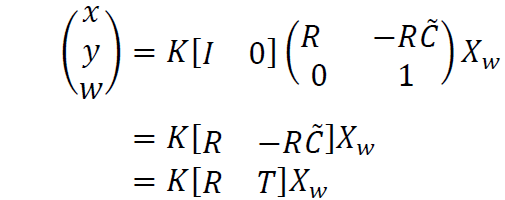其中：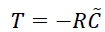[R T] 是一个3x4的矩阵，Xw是一个4x1的矩阵，K是一个3x3的矩阵。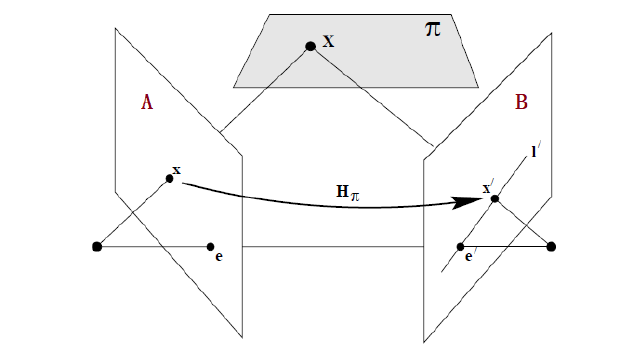图1 空间中同一点投影到两图像上

为了讨论更为一般的情况，我们假设两张图片来自于两个相机A、B(对应着左右图)，这样两个相机的内参矩阵是不同的，分别为Ka、Kb。世界坐标系中的三维点到相机坐标系转换，对应的旋转、平移矩阵分别为：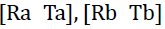这样一来，我们就可以得到两个相机模型矩阵。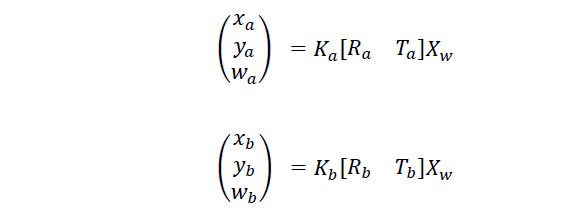将世界坐标系中的点用非齐次形式（头顶一飘~）表达为：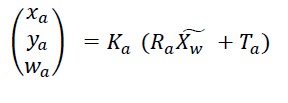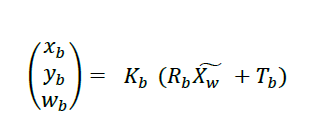1. 推导一：

在相机坐标系下，空间中的平面模型为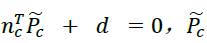是在相机坐标系下的非齐次坐标。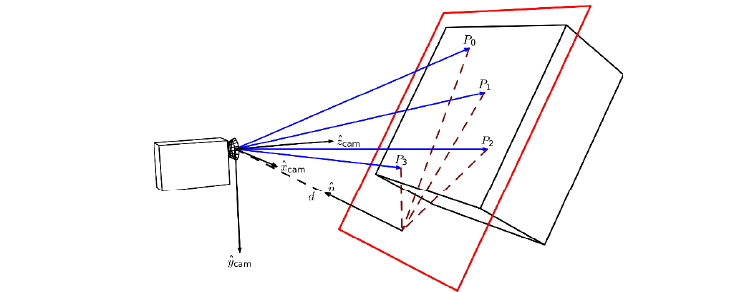图2 相机看空间中的一个平面

接下来推导从左图（相机A）到右图（相机B）的映射矩阵，需要用到的式子先摆上（下标中带字母“ca”和“a”，对应相机A）。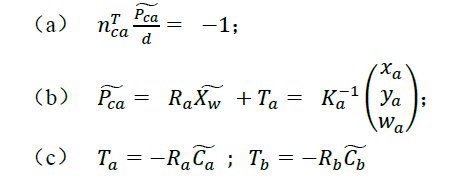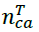是平面在相机A坐标系下的法向量，d表示该平面到相机A的垂直距离，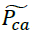是相机A坐标系下平面上的一点。

开启推导模式：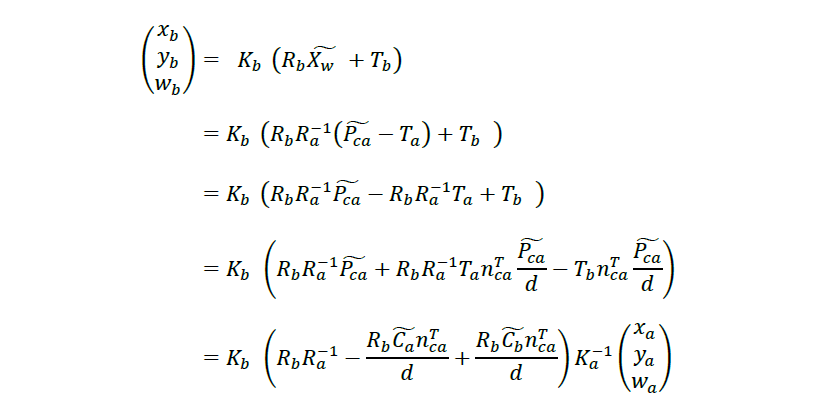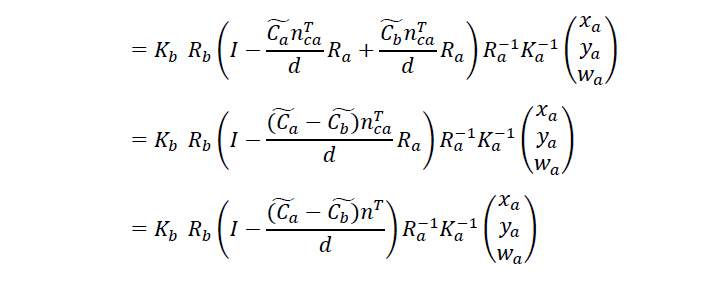需要说明一下：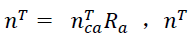是上述平面在世界坐标系中的法向量。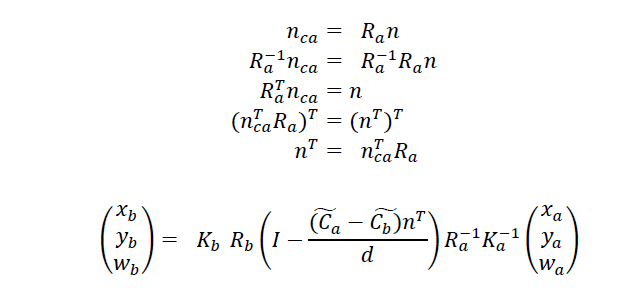旋转矩阵R是正交矩阵，所以R的转置就等于R的逆

至此，经过上述推导，两张图像中点的对应关系我们就得到了。

如果把世界坐标系和相机A坐标系重合，那么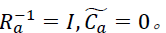上式化简为：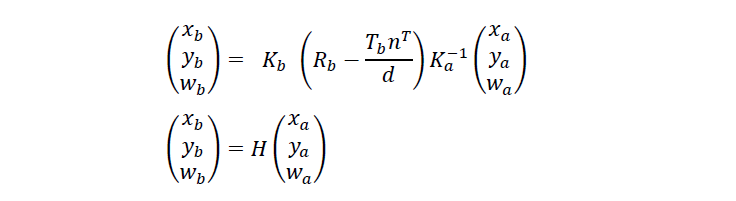单应矩阵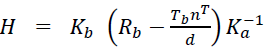H是一个3x3的矩阵，可以看出单应矩阵与两个相机之间的相对运动以及所拍摄平面特性有关系。

2. 推导二：

为了方便计算，假设所拍摄平面在世界坐标系中位于Z=0上。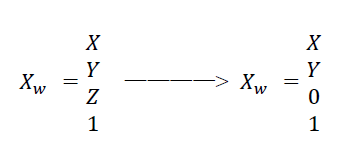这样Xw大小就由原来的4x1变成了3x1，旋转矩阵R中第三列就可以消去，[R T]变成了3x3的矩阵。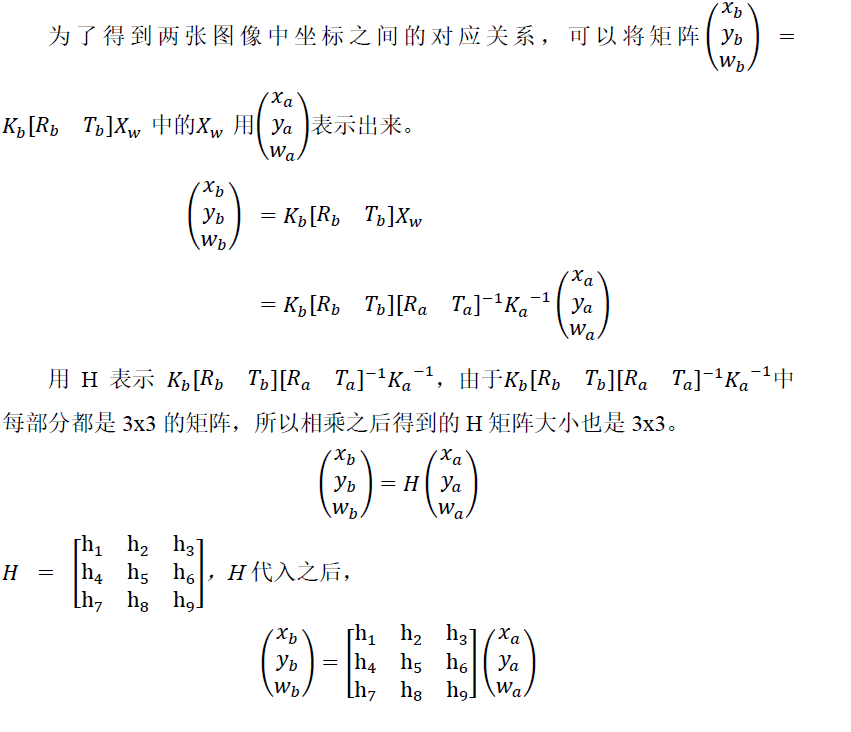3. 如何计算H: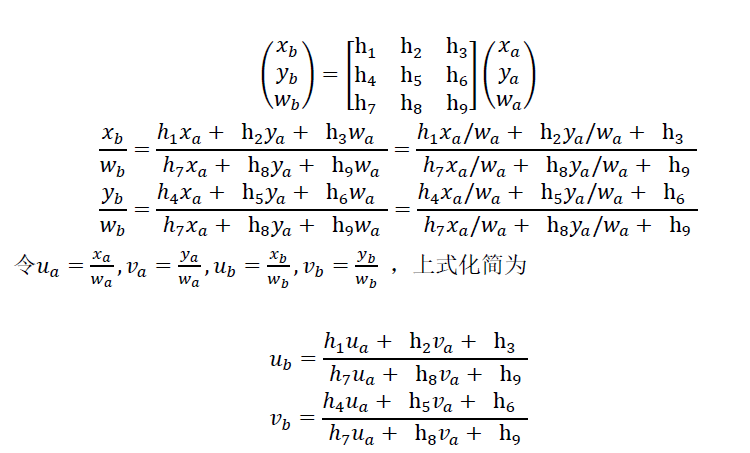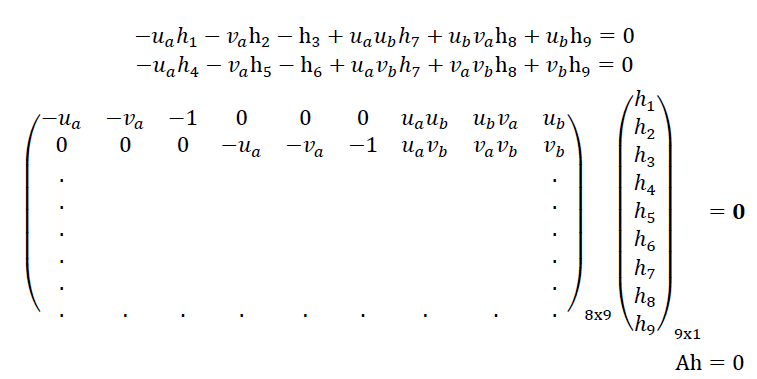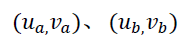为两张图像中匹配的点对（可以利用sift、surf等描述子匹配获得），每一对匹配点，可以得到两个方程。H矩阵中h9是尺度，所以一共有8个未知数，则至少需要4对点就可以解出矩阵H。解H矩阵常用的一个方法是SVD分解，以后我们会讲一下SVD分解。

这里给出MATLAB中求解H的代码：

[U,S,V] =svd(A);
H=V(:,end);
H=reshape(H,3,3);

至此，单应矩阵的推导以及计算就讲解完了。这里仍然需要强调的是：使用单应矩阵，需要确保三维点在一个平面上。（当然并不是必须在一个平面上，因为如果三维点距离相机比较远，三维点之间的距离和其距离相机的距离相比就很小，那就可以认为在一个平面上了。）

本文同步更新微信公众号：展开全文计算机视觉 homography
• ## 矩阵相关公式

万次阅读 2021-03-01 10:56:28
矩阵、列矩阵：m x n矩阵中，m=1的为矩阵。n=1的为列矩阵; 零矩阵：所有元素都为0的m x n矩阵; 方阵：m=n的m x n矩阵; 单位阵：主对角线上都为1,且其余为0 。n阶单位方阵称为E; 对角形矩阵：非对角线上的...卷积
• 维数组的地址计算 (m*n的矩阵) 优先 设每个元素的大小是size，首元素的地址是a，则a[i][j]? 分析：a[i][j]位于第i，第j。它之前有i-1，在第i它之前有j-1个元素。 即a[i][j] = a + [n*(i-1) ...
• 协方差矩阵的定义及其计算公式   (2014-03-20 18:36:56) 转载▼   分类： matlab 协方差矩阵在机器学习中经常用到，查看wiki：http://zh.wikipedia.org/wiki/协方差矩阵 可知...
• 协方差矩阵计算实例 突然发现给一组数据去实际计算对应得协方差矩阵，让人有点懵，并未找到太清楚的讲解，这里举一个实例记录一下。 1、别把样本数和维度数搞混了 具体进行计算容易懵的原因就是很容易把样本数和...协方差
• ## 二维卷积/矩阵卷积

万次阅读 多人点赞 2017-07-22 05:09:02
维卷积/矩阵卷积的计算方程设有矩阵A和矩阵B，它们的卷积结果矩阵的元素可由下列公式计算得来： C(j,k)=∑p∑qA(p,q)B(j−p+1,k−q+1)C(j,k)=\sum_p \sum_q A(p,q) B(j-p+1,k-q+1) 其中的index只要在A，B中valid...
• 维数组实验题：按如下公式递归计算矩阵行列式的值： 提示信息：“Please enter matrix size n(1<=n<20):” “Please input matrix line by line:\n” “matrix a:\n” **输出格式要求：“Submatrix:\n” ...c语言
• 本文针对长点数FFT计算开发了一种基于矩阵的FFT算法，此算法将需要计算的复数点序列抽象为一个矩阵进行处理，将大点数计算分割为多次小点数FFT计算，并且小点数之间的处理互不影响，进而可在多核处理器上...算法
• 0范数： 向量中非零元素的...范数：矩阵中每绝对值之和的最大值 范数：矩阵中每绝对值之和的最大值 详细研究请访问： 范数对于数学的意义？1范数、2范数、无穷范数_yangpan011的博客-CSDN博客_无穷范数 ...
• 两个样本点的马氏距离计算示例： Matlab计算协方差矩阵验算（矩阵a的代表属性，代表样本点）： 得到协方差矩阵后，我们就可以计算出v和x之间的马氏距离了： Matlab验算: 切记：协方差矩阵计算的是不同维度之间的...数据挖掘
• 3D变换矩阵：平移、缩放、旋转3D变换矩阵是一个4x4的矩阵，即由16个实数组成的维数组，在三维空间中，任何的线性变换都可以用一个变换矩阵来表示。本文介绍从变换矩阵中提取出平移、缩放、旋...计算机视觉 算法 matlab
• ## 矩阵论公式总结

千次阅读 2019-11-23 10:32:01
是一次,Jordan块是一, λ \lambda λ 减去的是1所以值为1, ②然后 ( λ − 1 ) 2 (\lambda-1)^{2} ( λ − 1 ) 2 是次,Jordan块是两两列,对角线值为1,因为是 ( λ − 1 ) (\lambda-1) ( λ − 1 ) ,对角...
• 通过矩阵求和与按求和两个示例介绍CUDA并行算法设计的思路，希望对大家有所帮助。很多公司CUDA工程师面试也会考察这个题目。 1. 矩阵求和 矩阵A[M][N]，B[M]; B[i] =A+A+...+A[N-1]; 串行代码...
• c语言中如何获取一个维数组的行列数？有两种方式： 1 维数组的行列数在定义的时候就是确定好... 2 动态获龋 对于type array[A][从键盘输入一个数组a,统计每的和。 例如： 4 5 4 5 4 2 2 3#include i...
• ## 协方差矩阵计算方法

万次阅读 多人点赞 2019-09-10 16:40:44
1. 协方差矩阵 X,YX,YX,Y是两个随机变量，X,YX,YX,Y的协方差Cov(X,Y)Cov(X,Y)Cov(X,Y)定义为： cov(X,Y)=E[(X−μx)(Y−μy)] cov(X,Y) = E[(X-\mu_x)(Y-\mu_y)] cov(X,Y)=E[(X−μx​)(Y−μy​)] 其中： E(X)=μx...
• 矩阵基础 (1). 优先和优先的问题 2016年08月18日 18:37:00 林微 阅读数：7351 ...
• 例题：编写程序，实现矩阵（33）的转置（即行列互换）。 例如，若输入下面矩阵： 1 2 3 4 5 6 7 8 9 则程序输出： 1 4 7 2 5 8 3 6 9 请勿改动主函数main与其它函数中的任何内容，仅在函数fun的花括号中填入所...c语言 程序设计 编程语言
• Kappa系数用于一致性检验，也可以用于衡量分类精度，但kappa系数的计算是基于混淆矩阵的. kappa系数是一种衡量分类精度的指标。它是通过把所有地表真实分类中的像元总数（N）乘以混淆矩阵对角线（Xkk）的和，再减去...kappa系数 matlab
• ## 协方差矩阵的计算及意义

万次阅读 多人点赞 2018-12-10 10:13:39
一、首先看一个比较简洁明了的协方差计算介绍： 1. 协方差定义 X、Y 是两个随机变量，X、Y 的协方差 cov...矩阵中的数据按排列与按排列求出的协方差矩阵是不同的，这里默认数据是按排列。即每一是一个obs...
• 矩阵求导公式的推导和计算引言全微分公式一个例子链式求导法则的应用 引言 在上一篇文章《机器学习中的向量求导和矩阵求导》中，我们介绍了关于矩阵求导和向量求导的一些定义和计算方式。这些内容对于理解向量和矩阵...机器学习 神经网络
• 线性代数在科学领域有很多应用的场景，如下：矩阵，是线性代数中涉及的内容，线性代数是用来描述状态和变化的，而矩阵是存储状态和变化的信息的媒介，可以分为状态(静态)和变化(动态)信息来看待。描述一个事物的状态...
• 矩阵是什么？ 我们都知道映射指的是一个空间 Rm\mathbb{R}^mRm到另一个空间 Rn\mathbb{R}^nRn的变换关系，狭义的函数其实是映射的一种特例，特指实数集间 R1\mathbb{R}^1R1的映射关系。 在所有映射中，我们最常见的...机器学习 深度学习
• 3*3矩阵与3*2矩阵乘法公式3*3矩阵与3*2矩阵相乘结果： AB=aA+bB+cC aD+bE+cF dA+eB+fC dD+eE+fF gA+hB+iC gD+hE+iF A=a b c d e f g h ...2*3矩阵与2*2矩阵乘积的详细解法两个矩阵相乘， 前者的数应当等于后者的...
• Ac是矩阵的线性组合，公式如下 上述公式在零空间、基、对角化等操作中具有重要作用！ 参考书籍： Sheldon Axler 《线性代数应该这样学》
• 这几天由于用到矩阵求导相关的知识，但是自己没有学过矩阵论(研究生选课的时候，导师没有让选)，于是百度了下，...矩阵求导 属于 矩阵计算，应该查找 Matrix Calculus 的文献：在网上看到有人贴了如下求导公式：Y...
• 系列简介：这个系列文章讲解线性代数的...) 关于向量与向量“左右乘”问题的详细介绍见下文： 线性代数入门——矩阵乘法满足的运算律及一些须要注意的问题 上一篇：线性代数入门——从线性变换的角度理解矩阵乘法...